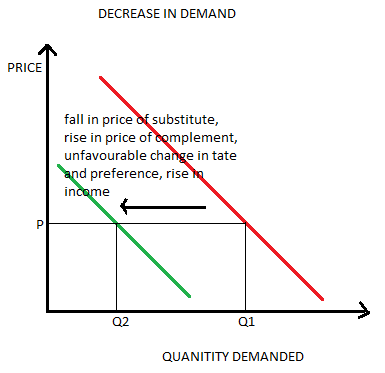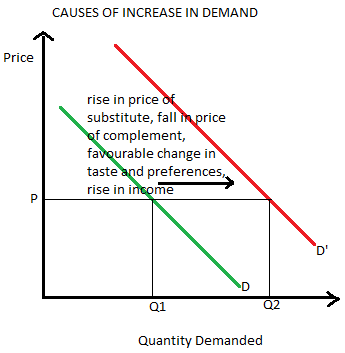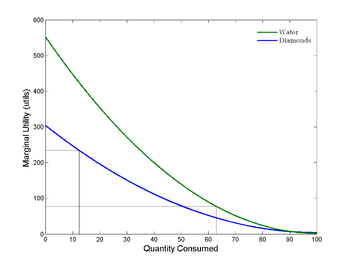# Microeconomics Discussion Questions

## 10 Most Important Questions in Microeconomics (with answers)

Online Microeconomics tutors at AssignmenthelpNet have created a list of 10 basic microeconomics discussion questions which every student of economics must know. This important microeconomics question list will have students to prepare for interviews, class tests, assignments and homework problems as well as to clearly understand important concepts of Microeconomics.

1. List four shift factors of demand and explain how each affects demand.

Ans: The four factors, which affect demand, are:

### Price of Substitute:

When the price of substitute decreases, people tend to buy more of the substitute and the demand of the product reduces. Thus, its demand curve shifts to the left. Similarly, when price of substitute rises, the consumer shifts his consumption to the product. Thus, its demand rises and demand curve shifts to the right. Example of substitutes is: tea and coffee.

### Price of complement

When price of one substitute falls, its demand falls. Thus, the demand for its complement also falls. Thus, the demand curve of the product shifts to the left. Similarly, when price of the complement rises, both the goods are jointly demanded in a greater quantity. Thus, the demand curve shifts to the right. An example of complementary goods is: car and petrol.

### Taste and preferences of consumers

If the consumers develop the taste and prefer a particular product, its demand rises and the demand curve shifts to the right. Similarly, when the consumers withdraw their preference from a good, its demand falls causing the demand curve to shift leftward.

### Income:

Normal good: Normal goods are those which the consumer considers superior. When the income of the consumer rises, he starts consuming more of normal goods. This makes its demand to rise and a rightward shift in the demand curve. On the other hand, a fall in the income causes the consumer to demand less of normal goods causing their demand to fall and thus, the demand curve shifts to the left.

Inferior good: These are the goods considered inferior to the normal goods. When the income rises, consumers buy less of inferior goods. Thus, its demand decreases causing leftward shift in demand curve. On the other hand, fall in income causes increased consumption of inferior goods. The increased demand causes the demand curve to shift rightward.2. In 1999, Domino's Pizza, a corporate sponsor of the Washington Redskins (a football team) offered to reduce the price of its medium-size pizza by \$1 for every touchdown scored by the Redskins during the previous week. Until that year, the Redskins weren't scoring many touchdowns. Much to the surprise of Domino's, in week one of 1999, the Redskins scored six touchdowns. As a result, the price of Domino's pizzas fell from \$8 a pie to \$2 a pie the following week. The quantity of pizzas demanded soared the following week from 1 pie an hour to 100 pies an hour. What was the price elasticity of demand for Domino's pizza?

Ans.

```{`
Q0 = 1
Q1 = 100
∆Q = Q1 - Q0 = 100-1 = 99
P0 = 8
P1 = 2
∆P = P1- P0 = 2-8 = -6
Price elasticity of demand for Domino’s pizza (Ep) = ∆Q/∆P * P0/Q0
= 99/(-6) * 8/1 = -132
`}```

Thus, price elasticity of demand is highly elastic, i.e., a small change in price causes a greater change in the quantity of pizzas demanded.

3. Early Classical economists found the following "diamond/water" paradox perplexing: "Why is water, which is so useful and so necessary, so cheap, when diamonds, which are so relatively unnecessary, are so expensive?" In modern economic terms, explain the water/diamond paradox.

Ans: According to the modern economic theory, the water diamond paradox can be explained on the basis of the marginal utility concept. Here the consumers are not choosing between all the diamonds in the world to all the water in the world; in such a case the water will be more valuable. They are just choosing between an additional unit of diamond to an additional unit of water, as the diamond is rarer than water thus diamond has a higher price.Water is available in a much abundant quantity than the availability of diamond. When the supply for water is less, the marginal utility derived from each unit of water is high as water is essential for survival. As this survival need is fulfilled, the marginal utility derived from water keeps on decreasing (according to the law of diminishing marginal utility).

Diamond is available less abundantly than water, thus, consumers are willing to pay higher price for diamonds.

However, if a person is dying of thirst, he will pay more for water than for diamonds because water is essential for him to survive. This is the concept of subjectivity.

Thus, it can be concluded that diamonds which are not a necessity are priced high due to their rarity while water, even after being a necessity is priced less because of its abundant availability.

4. Assume labour costs are 17.5% of revenue per vehicle for General Motors. In union negotiations during the late 1990s, GM attempted to cut its workforce to increase productivity. Together with the job reductions they planned, GM officials hoped to make the company's North American operations fully competitive with its U.S. and Japanese rivals with respect to total costs. Why are productivity gains so important to GM?

Ans. With tough competition from U.S. and Japanese firms, the profit margins and Brand Management Tutors of General Motors would be adversely affected. In order to improve its profitability and market share, GM has to focus on cost reduction. The current labour costs are as high as 17.5% of the revenue. Thus, it is important to curtail this cost. GMs attempt to cut down its workforce will lead to higher profitability and a better competitive stand in the market. The productivity (output per unit of input, here, labour) would also increase. Thus, the firm will get the benefits of productivity gains in the form of better profit margins, increased revenue and more competitiveness.

5. Your average total cost is \$40; the price you receive for a good is \$12. Should you keep on producing the good? Why?

Ans. Since the average total cost \$40 (ATC) is greater than the revenue received \$12 (AR), no profit is being earned; rather, there are high losses. Thus, the question here is to minimize losses as profits are in no case possible.

The decision depends on the level of average variable costs (AVC). So, we continue production as we are able to cover the variable costs and also some part of the fixed cost. If we discontinue production in this case, losses would equal the total fixed costs; however, if we continue production, the losses would be reduced to only that part of fixed cost that is not being covered. Thus, losses are minimized by continuing production.

If the average variable costs are higher than the revenue received (AVC > AR), we would discontinue production as not even the variable costs are being covered, let alone the fixed costs, thus, losses on operating would be the fixed cost as well as the uncovered portion of variable cost, which is higher than the losses if we discontinue production (i.e. fixed costs).

Suppose your average cost is \$40 in which your average fixed cost is \$30 but your average variable cost is only \$10. In this case by shutting down the firm will earn no revenue and would bear a loss equivalent to the entire average fixed cost per unit. So, the loss per unit will be \$30. However, if the firm continues to operate even when making loss, it will earn a revenue per unit of \$12. This per unit profit will be P-AVC which is \$12 - \$10 = \$2. Thus, the firm will be able to fully over its per unit variable cost while making a per unit profit of \$2. This \$2 profit will then be used to offset the loss on average fixed cost. Then, the net loss to the firm per unit will be Loss of Average Fixed Cost + Profit from operating under Loss = - \$30 + \$2 = -\$28. Evidently, by continuing to operate even while making loss in the short run the firm can effectively minimise its losses.

6. What portion of the marginal cost curve is the competitive firm's supply curve? How is a competitive firm's marginal cost curve related to the market supply curve?

Ans. The competitive firms supply curve is that part of the marginal cost curve (MC curve) that lies above the average variable cost curve (AVC curve). This is because a competitive firm produces when price equals MC equal MR above the Price = AVC level of output. This makes the supply curve equal to the marginal cost curve above the AVC level.

Since the market supply curve equals the horizontal summation of the short run individual supply curve of the competitive firms, thus, the market supply curve is the horizontal summation of the marginal cost curves (above the minimum AVC).

7. Monopolists differ from perfect competitors because monopolists always make a profit? True or false? Why?

Ans: The given statement is FALSE.

The monopolist makes profit always (both in short and long run); however, perfectly competitive firm can only earn profits in the short run and earns no economic profits in the long run. This is explained by the following major features of these market structures:

i. There is restricted entry in the monopoly market due to natural barriers or artificial barriers (patent rights, governmental regulations, etc.). This ensures that no new firms enter into the industry as the entry of new firms would lead to increased supply and thereby, a reduced price in the market. Thus, restricted entry ensures that the monopolist always earns profits. Under perfect competition, there is free entry and exit of firms. By seeing the firms making profits, new firms are induced to enter into the market. This increases the supply in the market causing prices to fall. This wipes out the supernormal profits and the firms earn only normal profit in the long run.

ii. The monopolist does not face any competition and thus, he is the price maker in the industry. On the other hand, under perfect competition, consumers don’t differentiate between different firms. As a result, firms have to accept the prices determined by the industry. Since the competitive firm is the price taker and not the price maker, it has is unable to raise prices or even reduce them. They are forced to abide by the industry price. Thus, in the long run, they do not earn profits as they cannot control or influence prices in the industry.

#### Need help with monopoly and Perfect Competition? Click hereto know more

8. What are the "monopolistic" and the "competitive" elements of monopolistic competition?

Ans. The monopolistic elements of a monopolistically competitive firm include:

• The firms have a substantial control over the price of their products (resembling a monopolist who is the price maker).
• The firms under both these market structures face a downward sloping demand curve or AR curve with the MR curve under it. (Indicating that they can sell more only by reducing the price).
• The firms in monopolistic competition can charge a price which is greater than marginal cost in both short run as well as in long run.

The competitive elements of a monopolistically competitive firm include:

• Similar to a perfectly competitive firm, there are many firms under the monopolistically competitive industry.
• There is freedom of entry of new firms and exit of existing firms under both these markets.
• In the short run, firms under both structures can earn losses or super-normal profits.
• In the long run, firms earn only normal profits that is they can only charge a price equal to their average cost. However, this price is not equal to the minimum average cost. Therefore, these firms are inefficient compared to perfect competition as they have excess capacity by not operating at the minimum point on the Average cost curve.

9. How is price elasticity of demand related to the tax revenue received from an imposed sales tax?

Ans: The tax revenue from imposed sales tax depends on the elasticity of demand of that product. If the demand of the product is inelastic then the revenue from sales tax will increase as the consumers will not change to a substitute and it would lead to an increase in tax revenue. On the other hand, if the demand for the product on which sales tax is imposed is elastic the consumers will change to a substitute product to avoid tax. The incidence of an ad-valorem tax such as a sales tax is also based on the elasticity of supply and demand. If the price elasticity of demand of a commodity is relatively elastic, then the consumer will bear lesser burden of the sales tax. However, if any good has relatively inelastic demand then the producer will be able to pass on a greater burden of the sales tax on to the consumer.